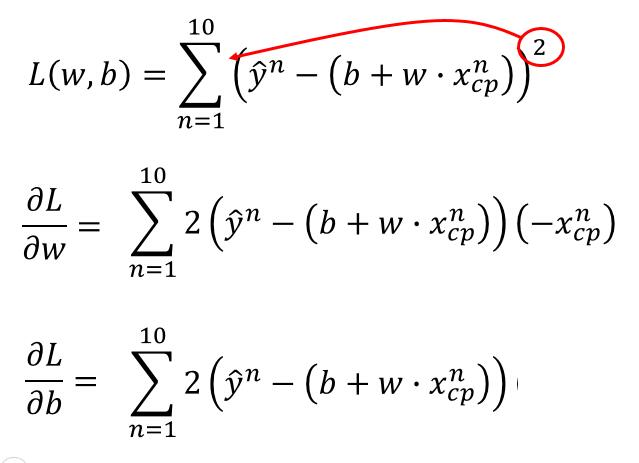Gradient Descent 美[ˈɡrediənt] [dɪˈsɛnt] : 梯度下降
Loss funcition 美 [lɔs] [ˈfʌŋkʃən] : 损失函数
Local Minima 美 [ˈloʊkl] [‘mɪnɪmə] : 局部最小值
Taylor series 美[ˈtelɚ] [ˈsɪriz] : 泰勒级数

## 一维变量

$$L = sin(w) + w^4 + w + log(w)+x_i$$
$x_i$是要输入的数据特征，例如上篇的 cp 值，可以看做常量。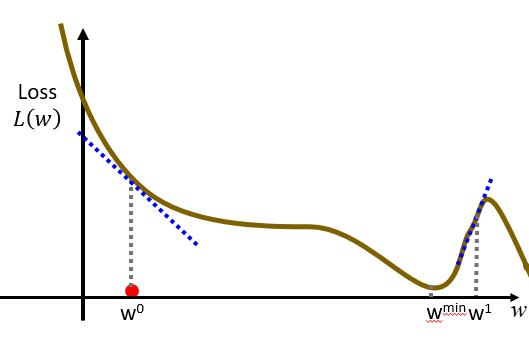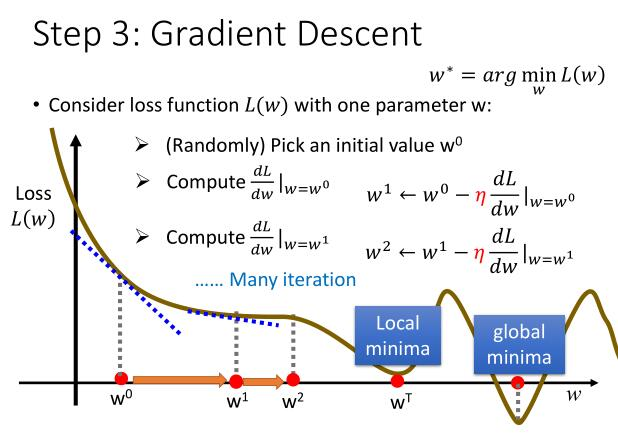## 二维变量

$$L = \theta_1 · x_1 + \theta_2 · x_2$$### Taylor series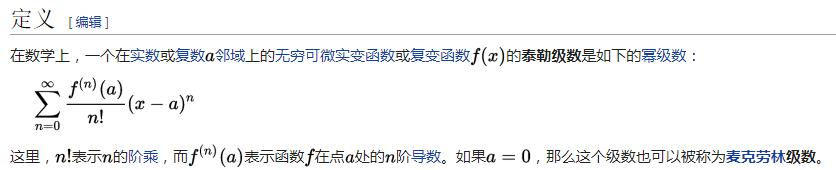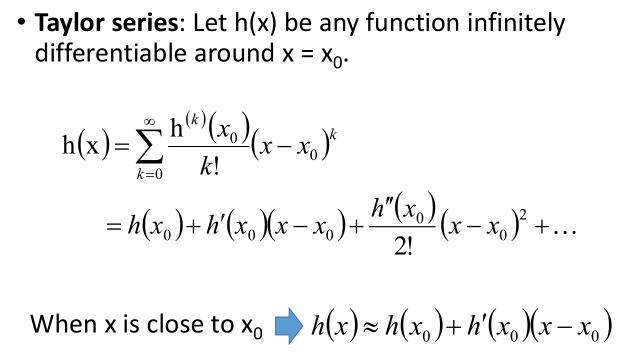### Multivariable Taylor Series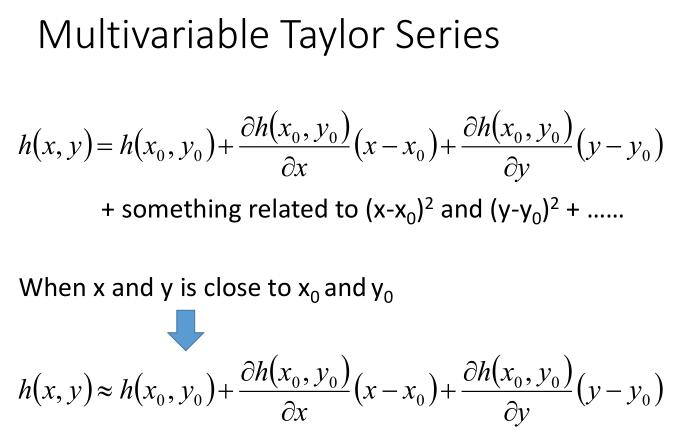### 推导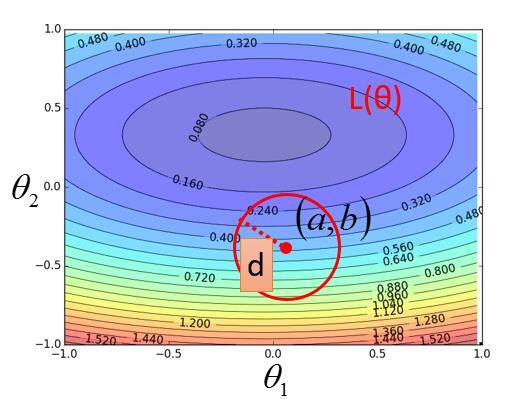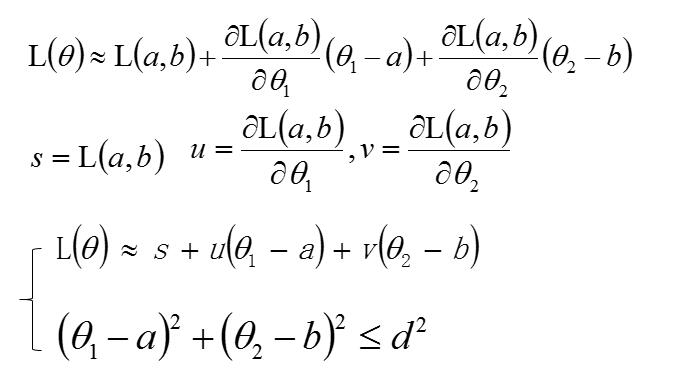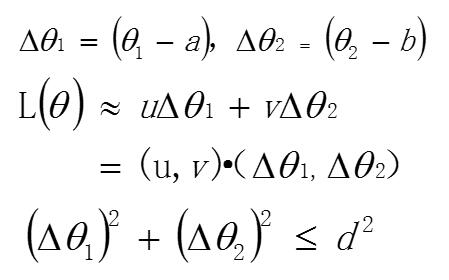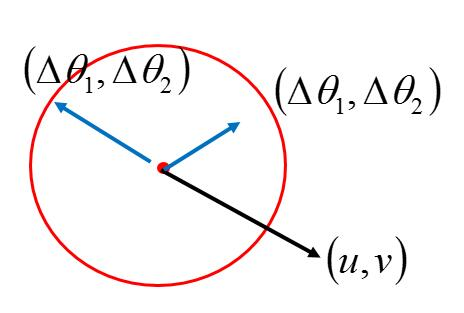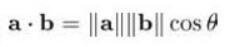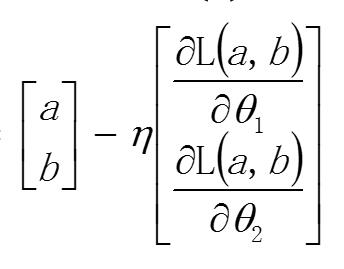！！！ 和一维变量一样，就是初始值，减去 $\eta$ 乘以他们的导数，但这里当然是偏导了。这样再回到开头，我们就先随便找一个初始值 (a , b)，利用这个公式然后一直迭代下去就可以了。

## 总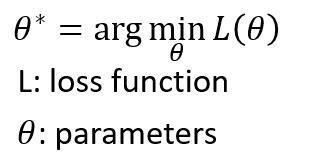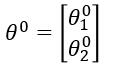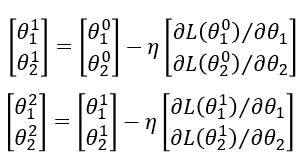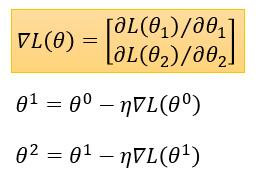## 实例

$$L = \sum_{n=0}^{10} ( \hat{y}^n - ( b+ w · x{^n_{cp}} ) )^2$$